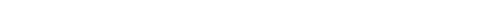## Question of the Week

 Shuffling an Array by kirupa  |  11 May 2011 Let's say you have an array whose data is the numbers 1 through 8 stored sequentially as visualized below:There may be times where you want to shuffle the contents of this array so that your array's data is more random. You may want to end up with something like this instead: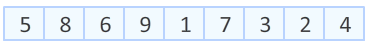In this short tutorial, let's look at the code for taking the contents of an array and shuffling it. Code for Shuffling an Array The approach I use for shuffling the contents of the array is to use something that Fisher-Yates devised and Don Knuth popularized. You'll see later towards the end of this article why that particular detail is important. Anyway, let's go ahead and look at the code: public function Main() { var tempArray:Array = [ 1, 2, 3, 4, 5, 6, 7, 8, 9, 10 ] ShuffleArray(tempArray); trace(tempArray); } public function ShuffleArray(input:Array) { for (var i:int = input.length-1; i >=0; i--) { var randomIndex:int = Math.floor(Math.random()*(i+1)); var itemAtIndex:Object = input[randomIndex]; input[randomIndex] = input[i]; input[i] = itemAtIndex; } } The ShuffleArray function, as its name implies, is responsible for shuffling the contents of your array. To use it in your code, simply call this function by passing it the array whose contents you wish to shuffle. After the ShuffleArray function has run, the array you passed in will be shuffled. Pretty simple. If all you wanted was the code to shuffle the contents of an array in ActionScript 3, you can pretty much stop here. If you wanted to learn a bit more about how the shuffling actually works, you should read on. If you had to ask me, I would strongly suggest you read on because I had a lot of fun writing it :P How the Shuffling Works Now that you have seen the code, let's take a step back and learn more about how our shuffling actually works. Let's start with the array that we started with:The first thing we do is start at the end of our array by selecting our last item:What we are going to do is swap the contents of our currently selected item with another item randomly selected from our array. The range of items I can randomly pick from are the selected item itself and all items that precede it: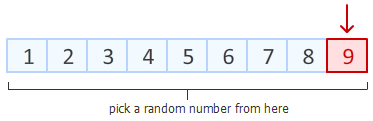Let's say that random item that we picked is the one containing the 4: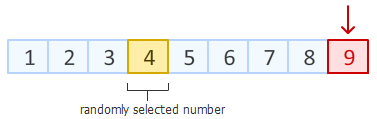Once the random number has been picked, we swap the contents of our selected item with the randomly selected item: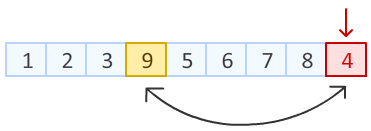With the swap completed, we are done with our last item. It is time to move on to the next item. Since we are going through our array backwards, that would be the item that preceded our last item: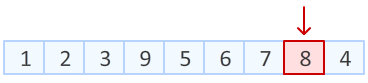Now, it is time for us to pick another item from our array to swap with this newly selected item. An important detail that I want to call out is that the range of numbers you can randomly pick from no longer includes the last item you addressed earlier: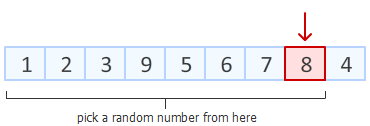The reason for why goes back to what I mentioned earlier - "The range of items I can randomly pick from are the selected item itself and all items that precede it." The random number picked in this iteration is the 2:Once the random item is picked, we peform the swap with our selected item. After the swap has been done, this whole process continues all over again by us moving on to the next (previous) item: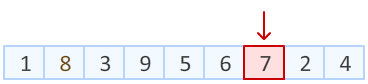At the risk of sounding too boring, I am not going to go any further by dissecting how the rest of the numbers will get shuffled. The two cases I described should prepare you well for playing out the remainder of the items. Now, let's shift our gears to take a look at how our code helps you to do what was just described. Looking at the CodeIn the preceding section, you got an overview of how our algorithm works. Let's now look at how all of that translates into code...starting with the ShuffleArray function: public function ShuffleArray(input:Array) { for (var i:int = input.length-1; i >=0; i--) { var randomIndex:int = Math.floor(Math.random()*(i+1)); var itemAtIndex:Object = input[randomIndex]; input[randomIndex] = input[i]; input[i] = itemAtIndex; } } The ShuffleArray function takes one argument, and that is the array that you wish to shuffle. Inside this function, this array will be called input. The first thing we will look at is the for loop: for (var i:int = input.length-1; i >=0; i--) { var randomIndex:int = Math.floor(Math.random()*(i+1)); var itemAtIndex:Object = input[randomIndex]; input[randomIndex] = input[i]; input[i] = itemAtIndex; } This loop is responsible for going through every item in your array and swapping it with a random number. Notice that the direction of this loop is backwards. You start at the end of your array (input.length - 1) and stop at the beginning. The current position you are in the loop is defined by the i variable. You can say that the i variable refers to the position of the selected item from our earlier example:The next step is for us to pick our random number: var randomIndex:int = Math.floor(Math.random()*(i+1)); var itemAtIndex:Object = input[randomIndex]; The randomIndex variable stores the random number mapping to an item's position that we are interested in. Notice that the maximum value of our random number is not the array's length, but instead, the current index position. This matches our expectation that the random number you can pick is either your selected element (i) or anything that precedes it. Once you have your random item position, you can get that item's contents by simply referring to it in your array: var itemAtIndex:Object = input[randomIndex]; At this point, your code corresponds to the following diagram:The red arrow corresponds to the position defined by i, and the item colored in yellow is your randomly selected item (itemAtIndex). Once you have your randomly selected item, all that is left to do is swap their values together: input[randomIndex] = input[i]; input[i] = itemAtIndex; The swapping is done by the above two lines where I first set the item at position i as the item our randomIndex position is pointing to. At this very moment, the contents of input[i] can be found both at the i position but also at the randomIndex position. This is only a temporary problem though.  Earlier, you created a copy of the contents at input[randomIndex] in your itemAtIndex variable. This means you can rectify this problem by setting assigning this copied value back to input[i]. In fact, that is actually what you do in the last line! ConclusionWohoo! You just finished learning how to shuffle the contents of an array. Shuffling algorithms are quite interesting because there are many ways to shuffle the contents. One thing to keep in mind is that some algorithms may seem to shuffle the values, but the shuffling may be heavily biased and uneven. You don't have to worry about that with the approach I described here, but if you want to see how easy it is to make a faulty assumption that seems correct, check out Jeff Atwood's post titled The Danger of Naïveté. Just a final word before we wrap up. If you have a question and/or want to be part of a friendly, collaborative community of over 220k other developers like yourself, post on the forums for a quick response!